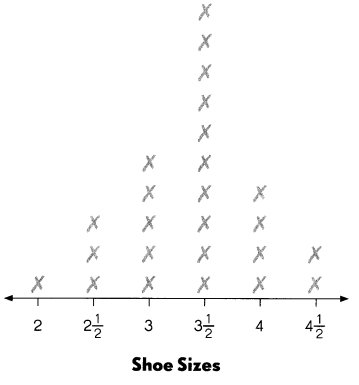# Math in Focus Grade 4 Chapter 5 Answer Key Data and Probability

Go through the Math in Focus Grade 4 Workbook Answer Key Chapter 5 Data and Probability to finish your assignments.

## Math in Focus Grade 4 Chapter 5 Answer Key Data and Probability

Math Journal

Write the steps to solve the problem.

Neil bought 5 books. The average price of 2 of the books is $5. The average price of the rest of the books is$4. Find the total amount of money Neil paid for the 5 books.

Then, following your steps above, solve the problem.

The total amount of money Neil paid for the 5 books is $16. Explanation: Given that Neil bought 5 books and the average price of 2 of the books is$5 and the average price of the rest of the books is $4. So the total amount of money Neil paid for the 5 books is as the price of 2 books is$5 and the price of 1 book is $4. So 5-2 is 3, and the price of the 4 books will be 4×3 which is$12. So the cost for 5 books it will be $12+$4 which is \$16.

Challenging Practice

Question 1.
Michelle got an average score of 80 on two tests. What score must she get on the third test so that her average score for the three tests is the same as the average score for the first two tests?
The score she got on the third test is 80.

Explanation:
Given that Michelle got an average score of 80 on two tests and the sum of score in 2 tests will be 80×2 which is 160. Let the score for third test be x,
so the new average will be $$\frac{160+x}{3}$$,
and the average score is 80, so $$\frac{160+x}{3}$$ = 80
160+x = 80×3
160+x = 240
x = 240 – 160
= 80.

Question 2.The line plot shows the shoe sizes of students in Ms. George’s class.

a. How many students are in the class?
25 students.

Explanation:
The total number of students are in the class is 25 students.

b. What is the mode of the set of data?
3$$\frac{1}{2}$$.

Explanation:
The mode of the set of data is 3$$\frac{1}{2}$$ as the number that appears most often.

c. How many students in the class wear a size 3$$\frac{1}{2}$$ shoe?
10 students.

Explanation:
The number of students in the class wear a size 3$$\frac{1}{2}$$ shoe is 10 students.

d. Suppose you looked at 100 pairs of shoes for the grade, which includes 3 other classes. How many pairs of size 3$$\frac{1}{2}$$ would there be? Explain your answer.

Problem Solving

Question 1.
The average height of Andy, Chen, and Chelsea is 145 centimeters. Andy and Chen are of the same height and Chelsea is 15 centimeters taller than Andy. Find Andy’s height and Chelsea’s height.
The Andy’s height and Chelsea’s height is 140 cm.

Explanation:
Given that the average height of Andy, Chen, and Chelsea is 145 centimeters and Andy and Chen are of the same height and Chelsea is 15 centimeters taller than Andy, so let the height of Andy and Chen be x and the height of Chelsea is 15 centimeters taller than Andy which is x+15. So Andy’s height and Chelsea’s height will be
$$\frac{x+x+x+15}{3}$$ = 145
$$\frac{3x+15}{3}$$ = 145
3x+15 = 145×3
3x+15 = 435
3x = 435-15
3x = 420
x = 420÷3
= 140.
So the Andy’s height and Chelsea’s height is 140 cm.

Question 2.
Eduardo has 3 times as many stamps as Sally. The average number of stamps they have is 450. How many more stamps does Eduardo have than Sally?
$$\frac{1}{2}$$ of total number of stamps extra.

Explanation:
Given that Eduardo has 3 times as many stamps as Sally and the average number of stamps they have is 450. Here, if Sally has 1 stamp then Eduardo has 3 stamps. So total stamps will be 4, Eduardo has 2 extra and Eduardo has $$\frac{2}{4}$$ which is $$\frac{1}{2}$$ of total number of stamps extra.

Question 3.
Bag A and Bag B each contain 2 marbles — 1 white and 1 red. Troy picks 1 marble from Bag A and 1 from Bag B. What is the probability that the following are picked?

a. 2 white marbles
$$\frac{2}{4}$$
The probability of picking up 2 white marbles is $$\frac{2}{4}$$.